# Voltage Drop Calculation Example

Here is an example of how to calculate voltage drop on a 12 Volt system. The result is the voltage drop at the end of each circuit.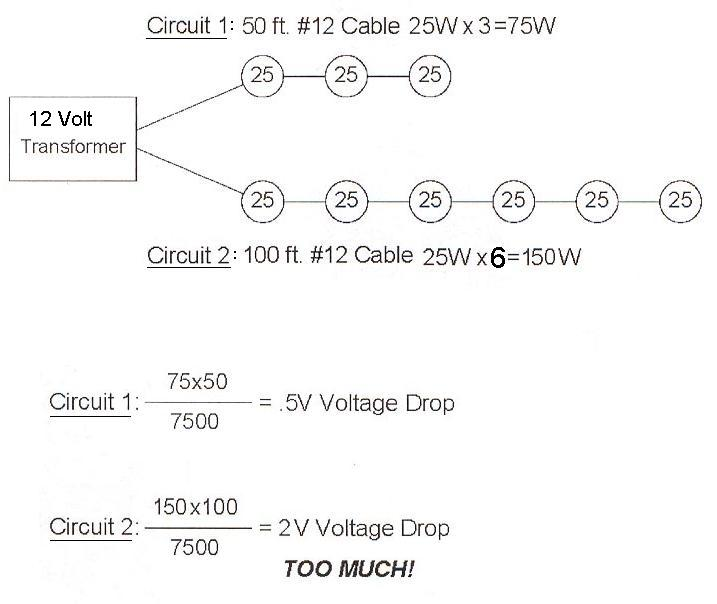Now, calculate the voltage drop on the same setup, but installed in the “T-splice” method. The distance to each end is still the same, but now you have two paths. Each path can be treated separately; you will also need to apply the 1.5 multiplier because most of the electrical load is on the far end of the cable run.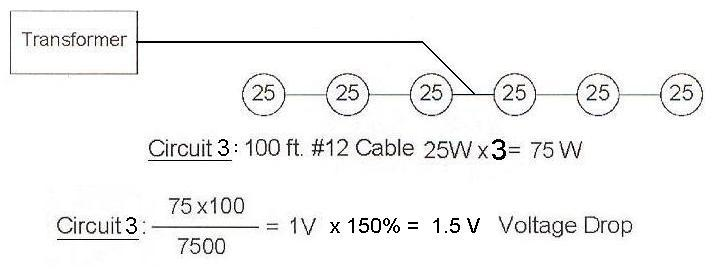So, the voltage at fixture C is 10.5 Volts. But 10.5 V is not preferred for halogen lamps, and say that is what you plan to use. If you need the fixtures to operate at higher voltages, then upgrade to a 10 gauge wire.

Here is the same 12 Volt setup with 10 gauge wire instead of 12 gauge.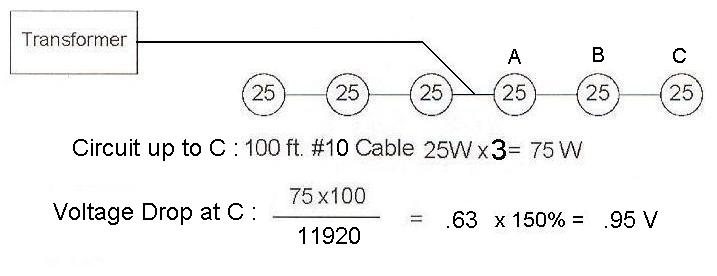This is a good amount of voltage drop for your halogen lamps. You can even calculate the voltage drop at individual fixtures. To solve the other voltage drops, you will need to know the distance from the power source to the fixture you are calculating. In the following illustration, assume the distance to the fixture labeled A is 80 feet.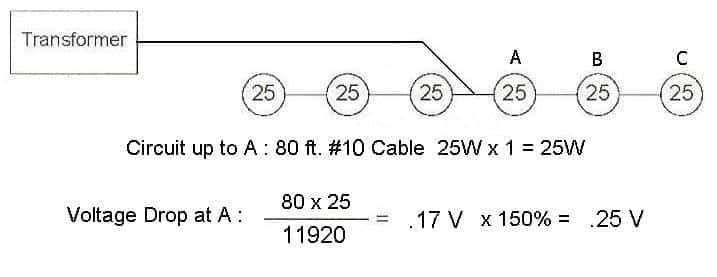The distance from fixture A to fixture B is 10 feet. Notice, the total wattage in our circuit includes all preceding fixtures.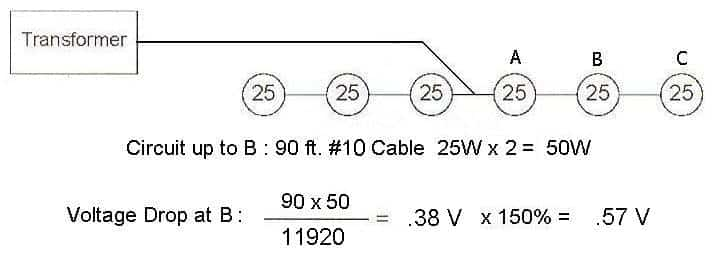So, the voltage at fixture A is 11.75 Volts, the voltage at fixture B is 11.43 Volts, and the voltage at fixture C is 11.05 Volts.